# Midpoint Formula

## Coordinate Geometry of Class 10

A Midpoint formula is used to determine the center point of a straight line. Sometimes you will need to find half of two specific numbers. Thus, you get the ratio of these two numbers. In the same way, we use the midpoint formula for coordinate geometry to find the average number (i.e., point) of two coordinates.

The coordinates of the midpoint M of a line segment AB with end points A(x1, y1) and B(x2, y2) are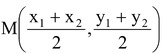.

Proof: Let M be the midpoint of the line segment joining the points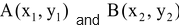Then M divides AB in the ratio 1 : 1.

So by the section formula, the coordinates of M are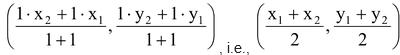Hence the coordinates of the midpoint of AB are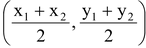.

Note: The line joining the points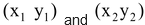is divided by the x-axis in the ratio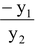and by x axis in the ratio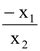To find the ratio in which the line divides the line joining the two points.

1. Let the ratio λ = 1.

2. Use the formula of internal division.

3. Evaluate the value of λ i.e., the ratio.

4. If λ is +ve then it is internal division and if λ is –ve then it is external division.

Q 1. Find the coordinates of the point which divides the line segment joining the points (6, 3) and (–4, 5) in the ratio 3 : 2 (i) internally (ii) externally.

Ans. Let P(x, y) be the required point.

(i) For internal division, we have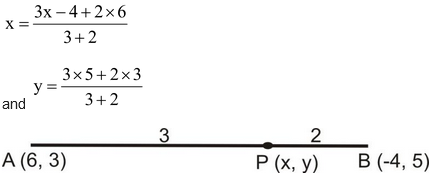⇒ x = 0 and y = 21/5.

So the coordinates of P are (0, 21/5).

(ii) For external division, we have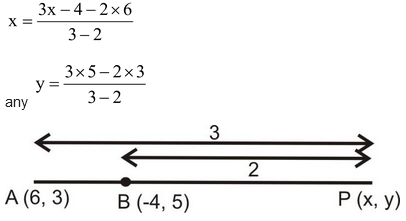⇒ x = - 24 and y = 9.

So the coordinates of P are (-24, 9).

Q2. In which ratio does the point (–1, –1) divide the line segment joining the pints (4, 4) and (7, 7)?

Ans. Suppose the point C(-1, -1) divides the line joining the points A(4, 4) and B(7, 7) in the ratio k : 1. Then, the coordinates of C are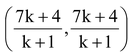.

But we are given that the coordinates of the points C are (-1, -1).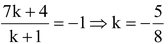Thus C divides AB externally in the ratio 5 : 8.

Q3. In what ratio does the X-axis divide the line segment joining the points (2, –3) and (5, 6)?

Ans. Let the required ratio be k : 1. Then the coordinates of the point of division are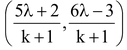. But it is a point on x-axis on which y-coordinate of every point is zero.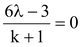⇒ k = 1/2.

Thus, the required ratio is 1/2 : 1 or 1 : 2.

Q1. What is the difference between mid point formula and section formula?

Ans. The midpoint of a line segment is the point that divides a line segment into two halves. The section formula builds on it and is a more powerful tool; it locates the point dividing the line segment in any desired ratio.

Q2. Who invented the midpoint formula?

Ans. Rene Descartes, born in 1596, invented the idea o geometrically representing ordered pairs of numbers. He was thrilled with his invention, which he called a method, for it used algebra to combine arithmetic and geometry and unified all the mathematics known up to that time.

Q3. What is the formula for finding the midpoint?

Ans. To find the midpoint of any range, add the two numbers together and divide by 2. In this instance, 0 + 5 = 5, 5 / 2 = 2.5

Q4. Why is the midpoint formula essential?

Ans. The midpoint formula is used when a person is required to find a straight center position between two defined points. So with the part of the line, use this formula to calculate the point that divides the part of the line defined by two points.

Q5. Why does the midpoint formula work?

Ans. The Midpoint Formula does the same thing. If one X-value is at 2 and the other X-value is at 8, to find the X-value halfway between them, you add 2+8 and divide by 2 = 5. You would repeat the Y-values process to find the midpoint's Y-coordinate.